# OliNo

Renewable Energy

## IPLED Tube SMD60CM-8W-PW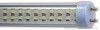presents a 60 cm long Tube light with 120 smd LEDs on it. The lamp shows to be very efficient. This article shows the measurement results.

Note that this lamp has been endurance tested.

Many parameters are also found in the Eulumdat file.

See this overview for a comparison with other light bulbs.

### Summary measurement data

parameter meas. result remark
Color temperature 5954 K Cold white.
Luminous intensity Iv 242 Cd Measured straight underneath the light bulb.
Beam angle 121 deg Valid for the C90-C270 plane, perpendicular to the tube’s length. For the C0-C180 the beam angle is 117 deg.
Power P 7.7 W
Power Factor 0.86 For every 1 kWh net power consumed, there has been 0.6 kVAhr for reactive power.
Luminous flux 740 lm
Luminous efficacy 96 lm/W What actually is meant here is luminaire efficacy, this accounts for all driver, thermal and luminair optical losses.
CRI_Ra 72 Color Rendering Index.
Coordinates chromaticity diagram x=0.3222 and y=0.3458
Fitting FL-tube
PAR-value 2.1 μMol/s/m2 The number of photons seen by an average plant when it is lit by the light of this light bulb. Value valid at 1 m distance from light bulb.
S/P ratio 2.0 This factor of higher efficacy when used in very low light circumstances.
D  x L external dimensions 28 x 590 mm External dimensions of the light bulb. Length without pins.
L x W luminous area 565 x 19 mm Dimensions of the luminous area (used in Eulumdat file). This is equal to the surface of the place the leds are mounted on.
General remarks The ambient temperature during the whole set of measurements was 24-25.5 deg C.

Warm up effect: during the warm up time the illumination and consumed power vary less than 5 %.

Voltage dependency: the power consumption and light output do not depend on the voltage applied. At the end an additional photo is given

Measurement report (PDF)Eulumdat fileRight click on icon and save the file.

### Overview tableThe illuminance values are measured in the far field (i.e. at least 5 times the distance of the largest illumination dimension) such that the light bulb can be seen as a point source. From this distance the values are calculated to values at the given distances.

If the light is not anymore a point source at any given distance, then the real measured values (with an actual lux meter) will be lower than indicated in this table. In general, the light source is not anymore a point source starting from distances lesss than 5x the length of the luminous area.

For this tube, this is starting from a distance of less than 5 x 565 mm = 2825 mm.

### Eulumdat light diagram

An interesting graph is the light diagram, indicating the intensity in the C0-C180 and the C90-C270 plane. This light diagram below comes from the program Qlumedit, that extracts these diagrams from an Eulumdat file.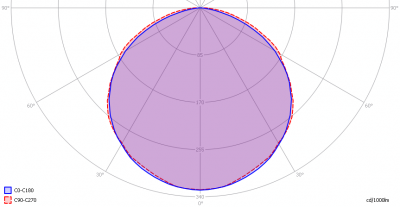The light diagram giving the radiation pattern.

It indicates the luminous intensity around the light bulb. This light bulb has an equal light diagram for the C0-C180 plane (in length direction of the tube) as for the C90-C270 plane (perpendicular to the length direction)

The unit is Cd/1000lm, meaning the intensity in Cd assuming there would be 1000 lumen in the measured light bulb. This enables comparing different types of light bulbs.

### Illuminance Ev at 1 m distance, or luminous intensity Iv

Herewith the plot of the averaged luminous intensity Iv as a function of the inclination angle with the light bulb.

The radiation pattern of the light bulb.

This radiation pattern is the average of the light output of the light diagram given earlier. Also, in this graph the luminous intensity is given in Cd. These averaged values are used (later) to compute the lumen output.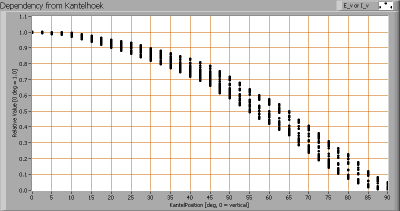Intensity data of every measured turn angle at each inclination angle.

This plot shows per inclination angle the intensity measurement results for each turn angle at that inclination angle. There normally are differences in illuminance values for different turn angles. However for further calculations the averaged values will be used.

When using the average values per inclination angle, the beam angle can be computed, being 117 degrees for the C0-C180 plane (in length direction of the tube) and 121 degrees for the C90-C270 plane (perpendicular to length direction).

### Luminous flux

With the averaged illuminance data at 1 m distance, taken from the graph showing the averaged radiation pattern, it is possible to compute the luminous flux.

The result of this computation for this light spot is a luminous flux of 740 lm.

### Luminous efficacy

The luminous flux being 740 lm, and the power of the light bulb being 7.7 W, yields a luminous efficacy of 96 lm/W.

A power factor of 0.86 means that for every 1 kWh net power consumed, a reactive component of 0.6 kVAr was needed.

 Light bulb voltage 230 VAC Light bulb current 39 mA Power P 7.7 W Apparent power S 9.0 VA Power factor 0.86

Of this light bulb the voltage across ad the resulting current through it are measured and graphed.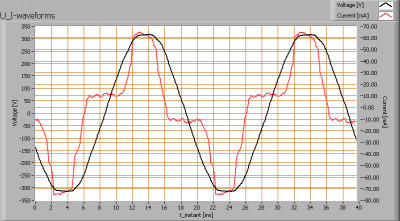Voltage across and current through the lightbulb

The current is almost in phase with the voltage. It has not a sinusiodal form. The number of steep edges in the current form will lead to some content of harmonics.

Also the power spectrum of the current is determined.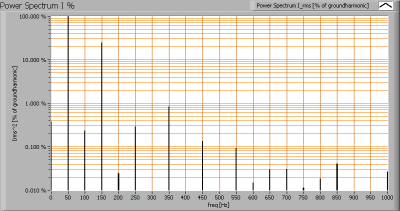Current power spectrum in % of the first harmonic (50 Hz).

Due to steep edges in the current as a result there are higher harmonics.

### Color temperature and Spectral power distribution

The spectral power distribution of this light bulb, energies on y-axis valid at 1 m distance.

The measured color temperature is about 6000 K which is cold white. This color temperature is measured straight underneath the light bulb.

Below a graph showing the color temperature for different inclination angles.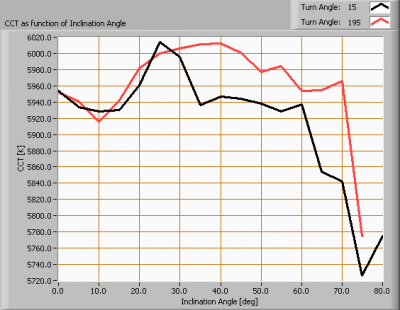Color temperature as a function of inclination angle.

At an inclination angle of 75 deg the illuminance was so low (less than 5 lux) that is stopped measuring color temperature.

The (maximum) beam angle is 121 degrees, meaning at an inclination angle of 60.5 degrees there is 50 % left of the initial illuminance value at 0 degrees. Most of the light energy is within this area. In this area the variation in light color is about 2 % of its value at 0 degrees inclination angle.

### PAR value and PAR spectrum

To make a statement how well the light of this light bulb is for growing plants, the PAR-area needs to be determined. PAR stands for Photosynthetic Active Radiation which is that radiation that participates actively to photosynthesis and is expressed in μMol/s/m2.

Photosynthesis is the essence for growth and flowering of plants, where the blue part of the light spectrum is responsible for growth and the red part is responsible for flowering and budding (for gemmation). For photosynthesis it is more important to count the number of photons rather than the power in the light. The power spectrum (power per wave length) of the light of the light bulb is converted into the number of photons (number of photons per wave length) and then these number of photons is weighted against the relative sensitivity of an average plant (which varies in value dependent on the wave length). The relative sensitivity curve in DIN-norm 5031-10:2000 is used for this.

The next image shows the result.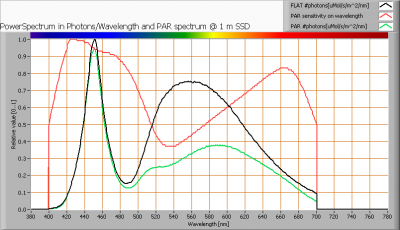The photon spectrum, then the sensitivity curve and as result the final PAR spectrum of the light of this light bulb

The black curve gives the power spectrum of the light of this light bulb, in number of photons per wave length. In red the relative sensitivity curve of an average plant (according to DIN norm 5031-10:2000). Multiplying these two results in a curve showing the number of photos per wave length of the light of this light bulb, the PAR spectrum.

Summing all the photons, gives a PAR number that for this light results in 2.1 μMol/s/m2. This value is valid at 1 m distance from the light bulb and in the area within the beam angle.

Dividing the PAR number by the total number of photons of the light of this light bulb gives a sort of PAR efficiency, which is 65 % (valid for the PAR wave length range of 400 – 700 nm). So maximally 65 % of the total of photons in the light is effectively used by the average plant (since the plant might not take 100 % of the photons at the frequency where its relative sensitivity is 100 %).

Note: when mentioning this percentage of efficiency, it is important to verify if all wavelengths are present in a sufficient manner. Not that only blue light is available, when the plant is going to be used for flowering where specifically red light is needed.

### S/P ratio

The human eye uses rods and cones. The rods work at scotopic light levels (twilight, night) and the rods at high(er) light levels (daytime, but also close to twilight).

Each type of receptor has its own sensitivity for light. The rods are 2.7 times more sensitive than cones, and most for light at a blueish-greenish color whereas compared to the cones’ sensitivity for green light (555 nm).

The S/P ratio gives the maximum difference in sensitivity of the rods at their most sensitive wavelength compared to the cones at their’s. The more blueish (cold white) the light, the more the S/P ratio can be.

The light of this light bulb has a certain spectrum leading to an S/P ratio of 2.0. The light of this lightbulb at scotopic light levels is perceived this factor higher than it would be a photopic light levels.

The power spectrum, sensitivity curves and resulting scotopic and photopic spectra (spectra energy content defined at 1 m distance).

It concerns a light bulb with cold white light, and therefore contains a lot of blue relative to red/green. Therefore the S/P ratio is high, being 2.0.

More explanation of the S/P ratio will come in a separate article.

### Chromaticity diagram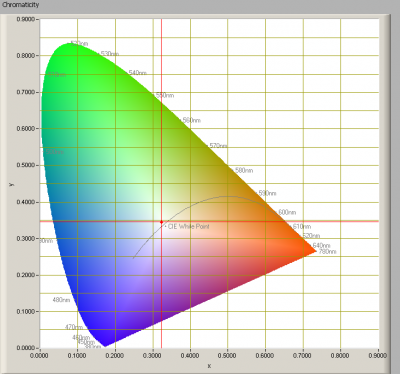The chromaticity space and the position of the lamp’s color coordinates in it.

The light coming from this lamp is somewhat distant to the Planckian Locus (the black path in the graph).

Its coordinates are x=0.3222 and y=0.3458.

### Color Rendering Index (CRI) or also Ra

Herewith the image showing the CRI as well as how well different colors are represented (rendered). The higher the number, the better the resemblance with the color when a black body radiator would have been used (the sun, or an incandescent lamp). Each color has an index Rx, and the first 8 indexes (R1 .. R8) are averaged to compute the Ra which is equivalent to the CRI.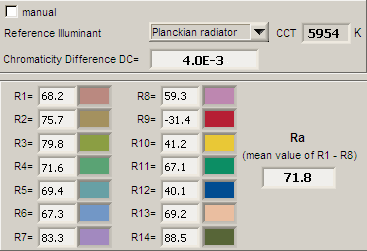CRI of the light of this lightbulb.

The value of 72 is lower than 80 which is considered a minimum value for indoor usage.

Note: the chromaticity difference is 0.004 indicates the distance to the Planckian Locus. Its value is lower than 0.0054, which means that the calculated CRI result is meaningful.

### Voltage dependency

The dependency of a number of lamp parameters on the lamp voltage is determined. For this, the lamp voltage has been varied and its effect on the following light bulb parameters measured: illuminance E_v [lx], the lamppower P [W], the (Correlated) Color Temperature [K] and the luminous efficacy [lm/W].Lamp voltage dependencies of certain light bulb parameters, where the value at 230 V is taken as 100 %.

The consumed power and illumination do not vary significantly when the voltage varies between 200-250 V.

When the voltage at 230 V varies with + and – 5 V, then the illuminance varies within 0.1 %, so when abrupt voltage changes occur this effect is not visible in the illuminance output.

### Warm up effects

After switch on of a cold lamp, the effect of heating up of the lamp is measured on illuminance E_v [lx], color temperature CT or correlated color temperature CCT [K], the lamppower P [W] and the luminous efficacy [lm/W].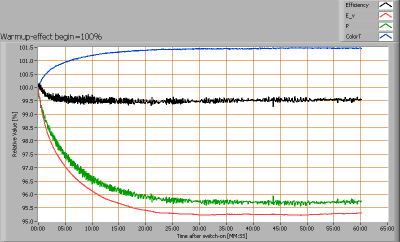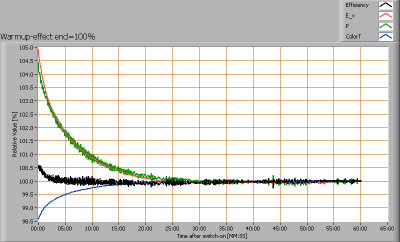Effect of warming up on different light bulb parameters. At top the 100 % level is put at begin, and at bottom at the end.

The consumped power and the illuminance vary less than 5 % during the warm up time of 25 minutes.